# 深度学习炼丹-数据标准化 原创

0收藏

## 前言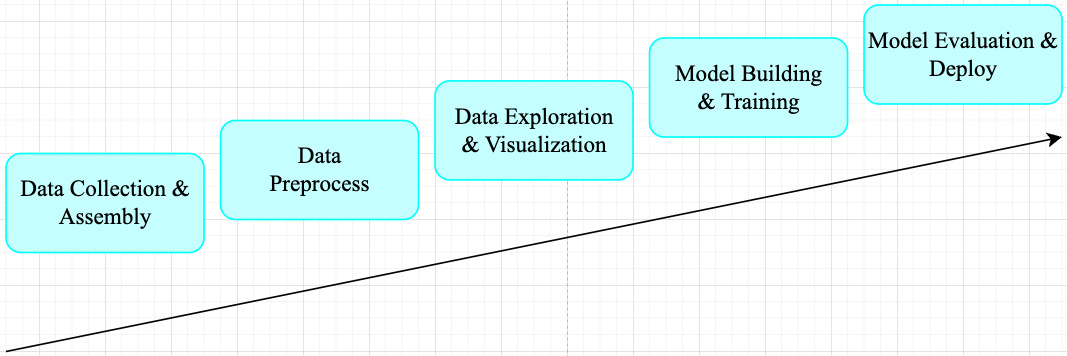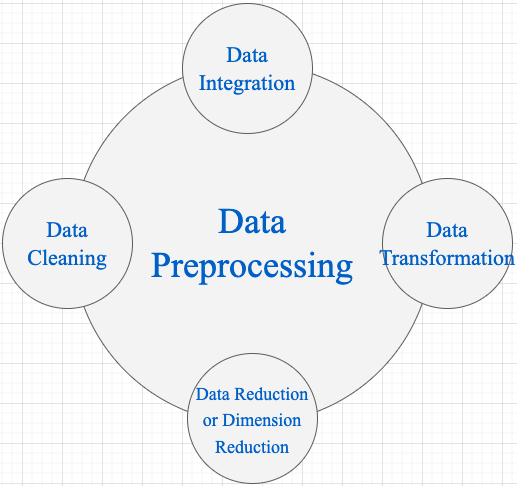1，Data Cleaning： 数据清理是数据预处理步骤的一部分，通过填充缺失值、平滑噪声数据、解决不一致和删除异常值来清理数据。

2，Data Integration： 用于将存在于多个源中的数据合并到一个更大的数据存储中，如数据仓库。例如，将来自多个医疗节点的图像整合起来，形成一个更大的数据库。

3，Data Transformation  : 在完成 Data Cleaning 后，我们需要使用以下数据转换策略更改数据的值、结构或格式。

• Generalization: 使用概念层次结构将低级或粒度数据转换为高级信息。例如将城市等地址中的原始数据转化为国家等更高层次的信息。
• Normalization: 目的是将数字属性按比例放大或缩小以适合指定范围。Normalization 常见方法:
• Min-max normalization
• Z-Score normalization
• Decimal scaling normalization

4，Data Reduction 数据仓库中数据集的大小可能太大而无法通过数据分析和数据挖掘算法进行处理。一种可能的解决方案是获得数据集的缩减表示，该数据集的体积要小得多，但会产生相同质量的分析结果。常见的数据缩减策略如下:

• Data cube aggregation
• Dimensionality reduction: 降维技术用于执行特征提取。数据集的维度是指数据的属性或个体特征。该技术旨在减少我们在机器学习算法中考虑的冗余特征的数量。降维可以使用主成分分析（PCA）等技术来完成。
• Data compression: 通过使用编码技术，数据的大小可以显着减小。
• Discretization: 数据离散化用于将具有连续性的属性划分为具有区间的数据。这样做是因为连续特征往往与目标变量相关的可能性较小。例如，属性年龄可以离散化为 18 岁以下、18-44 岁、44-60 岁、60 岁以上等区间。

## 一，Normalization 概述

### 1.1，Normalization 定义

Normalization 操作被用于对数据属性进行缩放，使其落在较小的范围之内（即变化到某个固定区间中），比如 [-1,1] 和 [0, 1]，简单理解就是特征缩放过程。很多机器学习算法都受益于 Normalization 操作，比如:

• 通常对分类算法有用。
• 在梯度下降等机器学习算法的核心中使用的优化算法很有用。
• 对于加权输入的算法（如回归和神经网络）以及使用距离度量的算法（如 K 最近邻）也很有用。

### 1.2，什么情况需要 Normalization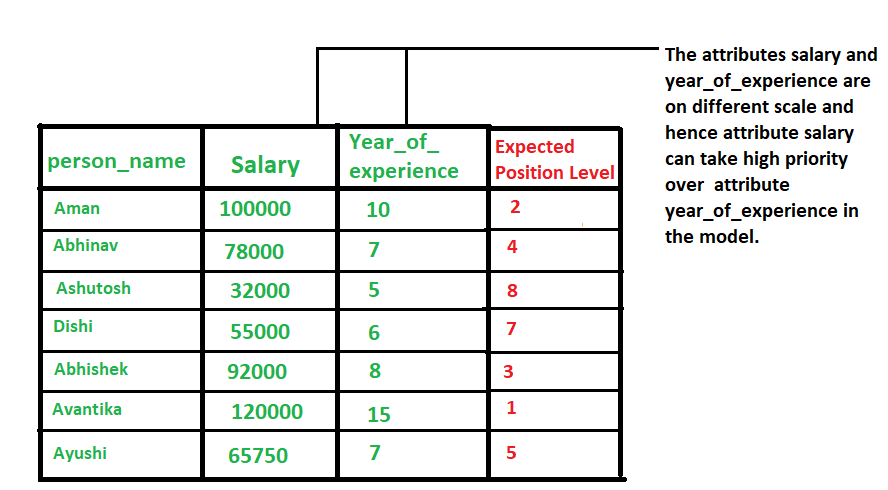### 1.3，为什么要做 Normalization

1，样本的各个特征的取值要符合概率分布，即 $[0,1]$（也可理解为降低模型训练对特征尺度的敏感度）。输入数据特征取值范围和输出标签范围一样，从损失函数等高线图来分析，不做 Normalization 的训练过程会更曲折。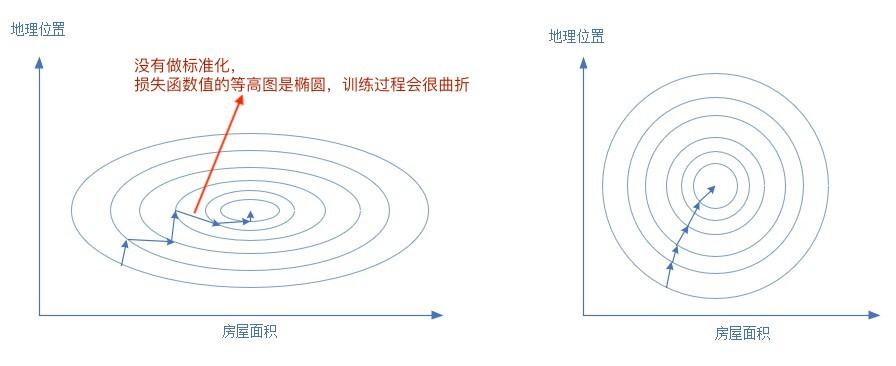2，神经网络假设所有的输入输出数据都是标准差为1，均值为0，包括权重值的初始化，激活函数的选择，以及优化算法的设计。

3，避免一些不必要的数值问题

4，梯度更新

5，学习率

### 1.4，Data Normalization 常用方法

1，z-Score Normalization

zero-mean Normalization，有时也称为 standardization，将数据特征缩放成均值为 0，方差为 1 的分布，对应公式:

$${x}’ = \frac{x-mean(x)}{\sigma}$$

• $x$：原始值
• $mean(x)$：表示变量 $x$ 的均值（有些地方用 $\mu =\frac{1}{N}\sum_{i=1}^{N} x_i$）
• $\sigma$: 表示变量的标准差（总体标准差数学定义 $\sigma = \sqrt{\frac{1}{N} \sum_{i=1}^{N}(x_i - \mu)^2}$ ）
• ${x}'$ 是数据缩放后的新值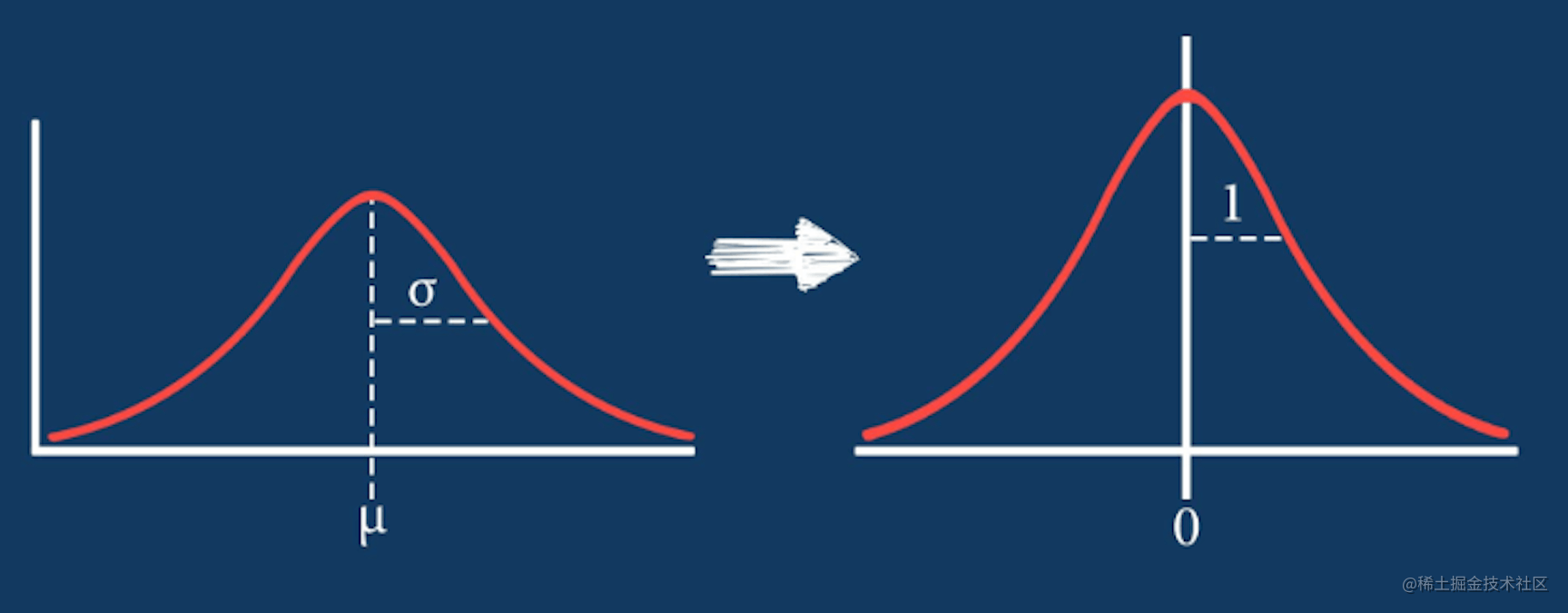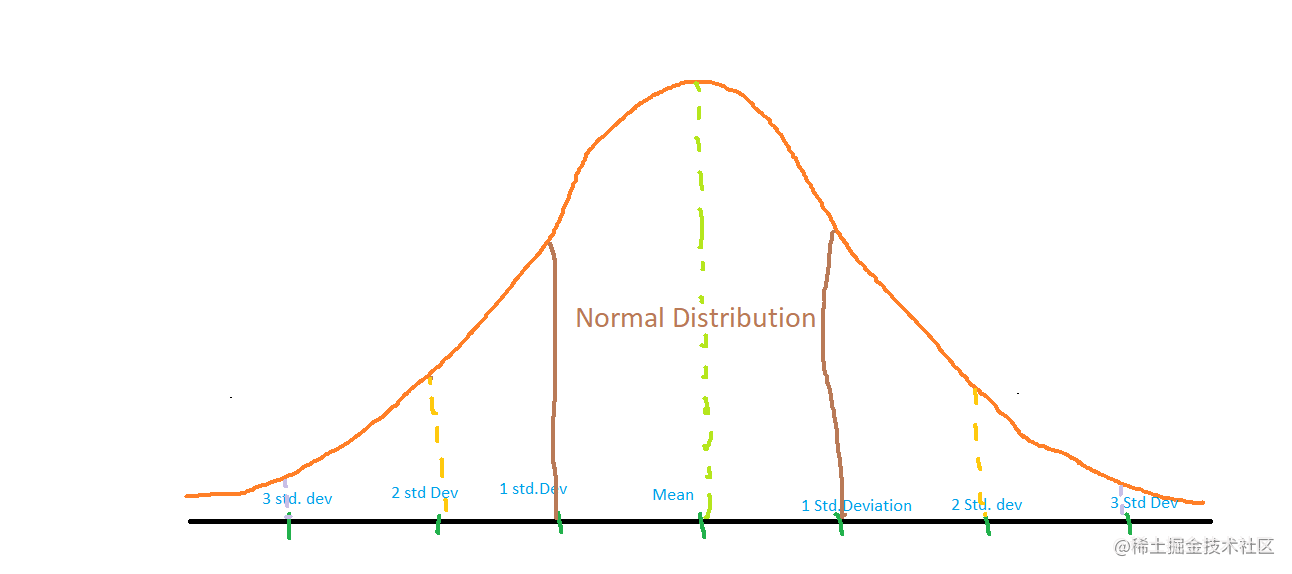2，Min-Max Normalization

$${x}’ = \frac{x - min(x)}{max(x) - min(x)}$$

### 1.5，代码实现

z-Score Normalization 方法既可以调用相关 python 库的 api 实现，也可以自己实现相关功能。

## 输出高清图像
%config InlineBackend.figure_format = 'retina'
%matplotlib inline
import numpy as np
from sklearn import preprocessing
import matplotlib.pyplot as plt
%matplotlib inline
import seaborn as sns

np.random.seed(42)

plt.figure(dpi = 200)
plt.figure(figsize=(20, 15))
# X_train = np.array([[ 10., -1.7,  21.4],
#                     [ 2.4,  0.,  0.6],
#                     [ 0.9,  1., -1.9]])
# 生成指定 size 和 范围 [low,high) 的随机浮点数
X_train = np.random.uniform(low=0.0, high=100.0, size = (100, 15))
# 1, 绘制原始数据的直方图
plt.subplot(3, 1, 1)
plt.title("original data distribution")
sns.distplot(X_train, color='y')

# 2， 应用 sklearn 库的 z-Score Normalization 类，并绘制直方图
scaler = preprocessing.StandardScaler().fit(X_train)
X_scaled = scaler.transform(X_train)
plt.subplot(3, 1, 2)
plt.title("z-Score Normalization by sklearn")
sns.distplot(X_scaled, color='r')

# 3，利用 numpy 函数实现 z-Score Normalization，并绘制直方图
def z_Score_Normalization(data):
data_mat = np.array(data)
data_z_np = (data_mat - np.mean(data_mat, axis=0)) / np.std(data_mat, axis=0)
return data_z_np

data_scaled = z_Score_Normalization(X_train)
plt.subplot(3, 1, 3)
plt.title("z-Score Normalization by numpy")
sns.distplot(data_scaled, color='g')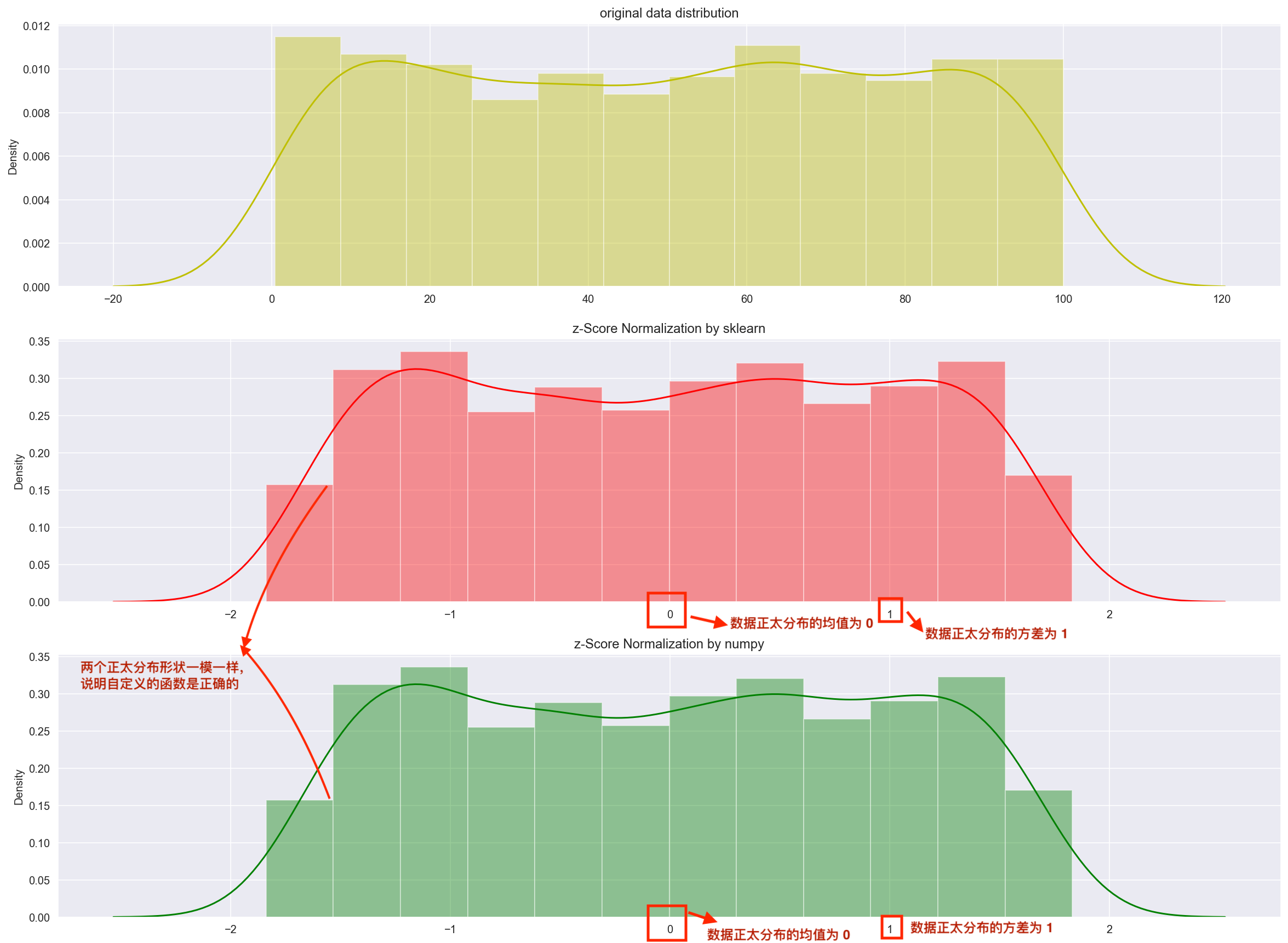Min-Max Normalization 方法的实现比较简单，以下是基于 numpy 库实现 Min-Max Normalization 功能的示例代码：

# 导入必要的库
import numpy as np
# 定义数据集
X = np.array([[1, 2, 3], [4, 5, 6], [7, 8, 9]])
def Min_Max_Normalization(X):
# 计算数据集的最小值和最大值
Xmin = X.min()
Xmax = X.max()
# 计算最小-最大规范化
X_norm = (X - Xmin) / (Xmax - Xmin)
return X_norm
# 打印结果
print(Min_Max_Normalization(X))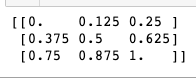## 二，normalize images

### 2.1，图像 normalization 定义

1，以单张图像的 zero-mean Normalization 为例，它使得图像的均值和标准差分别变为 0.01.0。因为是多维数据，与纯表格数据不同，它首先需要从每个输入通道中减去通道平均值，然后将结果除以通道标准差。因此可定义两种 normalization 形式如下所示:

# min-max Normalization
output[channel] = (input[channel] - min[channel]) / (max[channel] - min[channel])
# zero-mean Normalization
output[channel] = (input[channel] - mean[channel]) / std[channel]


### 2.3，PyTorch 实践图像 normalization

Pytorch 框架中，图像变换（image transformation）是指将图像像素的原始值改变为新值的过程。其中常见的 transformation 操作是使用 torchvision.transforms.ToTensor() 方法将图像变换为 Pytorch 张量（tensor），它实现了将像素范围为 [0, 255] 的 PIL 图像转换为形状为（C,H,W）且范围为 [0.0, 1.0] 的 Pytorch FloatTensor。另外，torchvision.transforms.normalize() 方法实现了逐 channel 的对图像进行标准化（均值变为 0，标准差变为 1）。总结如下:

• min-max Normalization: 对应 torchvision.transforms.ToTensor() 方法
• zero-mean Normalization: 对应 torchvision.transforms.Normalize() 方法，利用用均值和标准差对张量图像进行 zero-mean Normalization。

ToTensor() 函数的语法如下:

"""
Convert a PIL Image or numpy.ndarray to tensor.
Converts a PIL Image or numpy.ndarray (H x W x C) in the range
[0, 255] to a torch.FloatTensor of shape (C x H x W) in the range [0.0, 1.0].

Args:
pic (PIL Image or numpy.ndarray): Image to be converted to tensor.
Returns:
Tensor: Converted image.
"""


Normalize() 函数的语法如下:

Syntax: torchvision.transforms.Normalize()

Parameter:
* mean: Sequence of means for each channel.
* std: Sequence of standard deviations for each channel.
* inplace: Bool to make this operation in-place.
Returns: Normalized Tensor image.


1. 加载原图像；
2. 使用 ToTensor() 函数将图像转换为 Tensors；
3. 计算 Tensors 的均值和方差；
4. 使用 Normalize() 函数执行 zero-mean Normalization 操作。

# import necessary libraries
from PIL import Image
import matplotlib.pyplot as plt
import numpy as np
import torchvision.transforms as transforms
import matplotlib.pyplot as plt

def show_images(imgs, num_rows, num_cols, titles=None, scale=8.5):
"""Plot a list of images.

Defined in :numref:sec_utils"""
figsize = (num_cols * scale, num_rows * scale)
_, axes = plt.subplots(num_rows, num_cols, figsize=figsize)
axes = axes.flatten()
for i, (ax, img) in enumerate(zip(axes, imgs)):
try:
img = np.array(img)
except:
pass
ax.imshow(img)
ax.axes.get_xaxis().set_visible(False)
ax.axes.get_yaxis().set_visible(False)
if titles:
ax.set_title(titles[i])
return axes

def normalize_image(image_path):
img = Image.open(img_path) # load the image
# 1, use ToTensor function
transform = transforms.Compose([
transforms.ToTensor()
])
img_tensor = transform(img) # transform the pIL image to tensor
# 2, calculate mean and std by tensor's attributes
mean, std = img_tensor.mean([1,2]), img_tensor.std([1,2])
# 3, use Normalize function
transform_norm = transforms.Compose([
transforms.ToTensor(),
transforms.Normalize(mean, std)
])
img_normalized = transform_norm(img) # get normalized image

img_np = np.array(img) # convert PIL image to numpy array
# print array‘s shape mean and std
print(img_np.shape) # (height, width, channel), (768, 1024, 3)
print("mean and std before normalize:")
print("Mean of the image:", mean)
print("Std of the image:", std)
return img_np, img_tensor, img_normalized

def convert_tensor_np(tensor):
img_arr = np.array(tensor)
img_tr = img_arr.transpose(1, 2, 0)
return img_tr

if __name__ == '__main__':
img_path = 'Koalainputimage.jpeg'
img_np, img_tensor, img_normalized = normalize_image(img_path)
# transpose tensor to numpy array and shape of (3,,) to shape of (,,3)
img_normalized1 = convert_tensor_np(img_tensor)
img_normalized2 = convert_tensor_np(img_normalized)
show_images([img_np, img_normalized1, img_normalized2], 1, 3, titles=["orignal","min-max normalization", "zero-mean normalization"])


1，程序输出和两种 normalization 操作效果可视化对比图如下所示: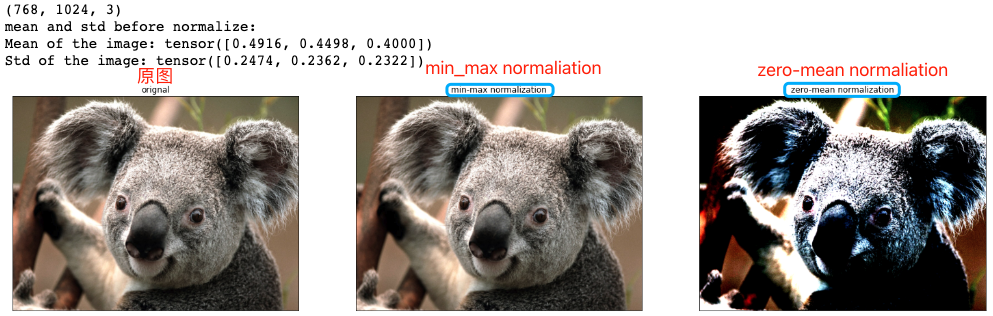2，原图和两种 normalization 操作后的图像像素值分布可视化对比图如下所示: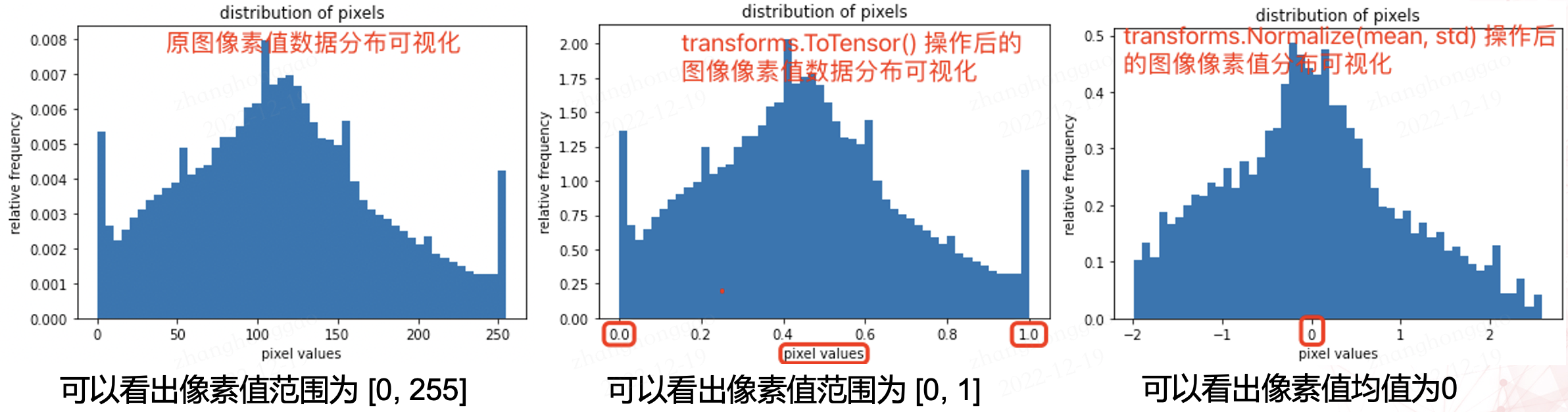# plot the pixel values
plt.hist(img_np.ravel(), bins=50, density=True)
plt.xlabel("pixel values")
plt.ylabel("relative frequency")
plt.title("distribution of pixels")


## 参考资料